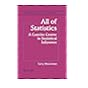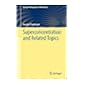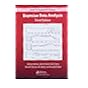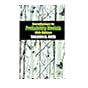Normal view

# Bayesian data analysis

Material type:TextLanguage: English Publisher: Boca Raton, Fla. [u.a.] CRC Press/Chapman & Hall c 2014Edition: 3. edDescription: XIV, 661 S. graph. Darst. 24 cmISBN: 1439840954 (hbk.); 9781439840955 (hbk.)DDC classification: 519.5/42 | MAT029000 LOC classification: QA279.5Other classification: 31.73 | 83.03 | QH 233 | mat Online resources: Zentralblatt MATH Inhaltstext | Inhaltsverzeichnis Inhaltsverzeichnis | Cover Summary: "Preface This book is intended to have three roles and to serve three associated audiences: an introductory text on Bayesian inference starting from first principles, a graduate text on effective current approaches to Bayesian modeling and computation in statistics and related fields, and a handbook of Bayesian methods in applied statistics for general users of and researchers in applied statistics. Although introductory in its early sections, the book is definitely not elementary in the sense of a first text in statistics. The mathematics used in our book is basic probability and statistics, elementary calculus, and linear algebra. A review of probability notation is given in Chapter 1 along with a more detailed list of topics assumed to have been studied. The practical orientation of the book means that the reader's previous experience in probability, statistics, and linear algebra should ideally have included strong computational components. To write an introductory text alone would leave many readers with only a taste of the conceptual elements but no guidance for venturing into genuine practical applications, beyond those where Bayesian methods agree essentially with standard non-Bayesian analyses. On the other hand, we feel it would be a mistake to present the advanced methods without first introducing the basic concepts from our data-analytic perspective. Furthermore, due to the nature of applied statistics, a text on current Bayesian methodology would be incomplete without a variety of worked examples drawn from real applications. To avoid cluttering the main narrative, there are bibliographic notes at the end of each chapter and references at the end of the book"--
Tags from this library: No tags from this library for this title.Average rating: 0.0 (0 votes)
Item type Current location Call number Status Date due Barcode Item holdsBook Library
519 (Browse shelf) Checked out 07/12/2020 AT-ISTA#000879
Total holds: 0
##### Close shelf browser519 All of Statistics: A Concise Course in Statistical Inference (Springer Texts in Statistics). 519 Convex Optimization Theory 519 Superconcentration and Related Topics 519 Bayesian data analysis 519 Introduction to probability models 519 Introduction to probability models 519 Introduction to probability models

Literaturverz. S. 607 - 639

"Preface This book is intended to have three roles and to serve three associated audiences: an introductory text on Bayesian inference starting from first principles, a graduate text on effective current approaches to Bayesian modeling and computation in statistics and related fields, and a handbook of Bayesian methods in applied statistics for general users of and researchers in applied statistics. Although introductory in its early sections, the book is definitely not elementary in the sense of a first text in statistics. The mathematics used in our book is basic probability and statistics, elementary calculus, and linear algebra. A review of probability notation is given in Chapter 1 along with a more detailed list of topics assumed to have been studied. The practical orientation of the book means that the reader's previous experience in probability, statistics, and linear algebra should ideally have included strong computational components. To write an introductory text alone would leave many readers with only a taste of the conceptual elements but no guidance for venturing into genuine practical applications, beyond those where Bayesian methods agree essentially with standard non-Bayesian analyses. On the other hand, we feel it would be a mistake to present the advanced methods without first introducing the basic concepts from our data-analytic perspective. Furthermore, due to the nature of applied statistics, a text on current Bayesian methodology would be incomplete without a variety of worked examples drawn from real applications. To avoid cluttering the main narrative, there are bibliographic notes at the end of each chapter and references at the end of the book"--

There are no comments for this item.